COLUMNS Function

Return the number of columns in a given array or reference

How to get excel last value in column

The COLUMNS Function in Excel is a Lookup/Reference function. It is useful for looking up and providing the number of columns in a given reference or array. The COLUMNS function, after receiving an Excel range, will return the number of columns that are contained within that range.

Formula

=COLUMNS(array)

The COLUMNS function in Excel includes only one argument – array. The array argument is a required argument. It is the reference to a range of cells or array or array formula for which we want the number of columns. The function returns a numerical value.

How to use the COLUMNS Function in Excel?

It is a built-in function that can be used as a worksheet function in Excel. To understand the uses of this function, let’s consider a few examples:

Example 1

Let’s see how this function works when we provide following references: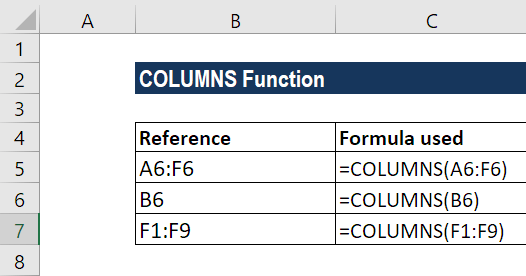Suppose we wish to find out the number of columns in a range then this function is useful. The most basic formula used is = COLUMNS(rng).

In the first reference above, we used the COLUMNS function to get the number of columns from range A6:F6. We got the result as 6 as shown in the screenshot below: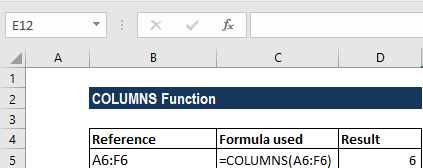So, this function counted the number of columns and returned numerical value as result.

When we gave the cell reference B6, it returned the result of 1 as only one reference was given.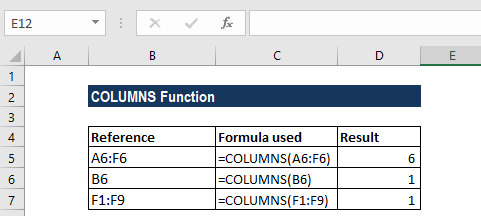Lastly, when we provided the formula F1:F9, I counted the number of columns as 1 and the function returned the result accordingly.

Thus, in this function, “array” can be an array, an array formula, or a reference to a single contiguous group of cells.

Example 2

Using Columns with other formulas. If we wish to get the address of the first cell in a named range, we can use the ADDRESS function together with ROW and COLUMN functions. The formula to be used is below: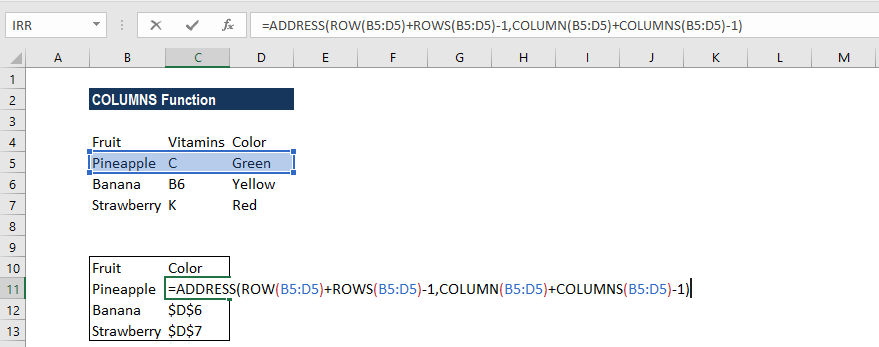What happens in this formula is that the ADDRESS function builds an address based on a row and column number. Then, we use the ROW function to generate a list of row numbers, which are then shifted by adding ROWS(B5:D5)-1, so that the first item in the array is the last row number:

ROW(B5:D5)+ROWS(B5:D5)-1

We do the same for COLUMN and COLUMNS: COLUMN(B5:D5)+COLUMNS(B5:D5)-1

What happens is the ADDRESS function now collects and returns an array of addresses. If we enter the formula in a single cell, we just get the item from the array, which is the address corresponding to the last cell in a range.

Example 3

Now let’s see how to find out the last column in a range. The data given is as follows: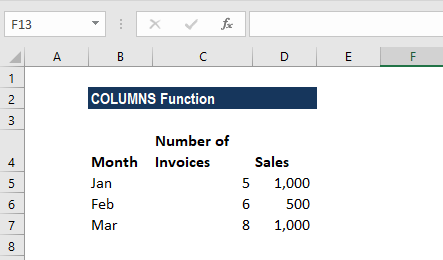The formula used is =MIN(COLUMN(A3:C5))+COLUMNS(A3:C5)-1

Using the formula above, we can get the last column that is in a range with a formula based on the COLUMN function.

When we give a single cell as a reference, the COLUMN function WILL return the column number for that particular reference. However, when we give a range that contains multiple columns, the COLUMN function will return an array that contains all column numbers for the given range.

If we wish to get only the first column number, we can use the MIN function to extract just the first column number, which will be the lowest number in the array.

Once we get the first column, we can just add the total columns in the range and subtract 1, to get the last column number.

The result is shown below: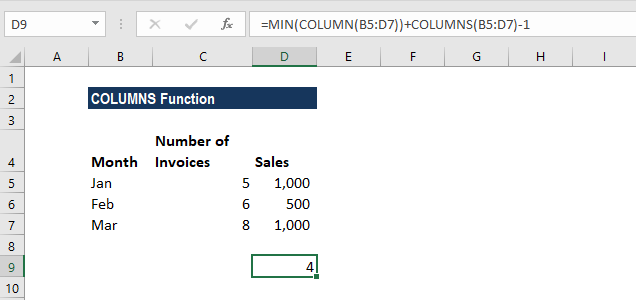For a very large number of ranges, we can use the INDEX function instead of the MIN function. The formula would be:

=COLUMN(INDEX(range,1,1))+COLUMNS(range)-1

Thanks for reading CFI’s guide to important Excel functions! By taking the time to learn and master these functions, you’ll significantly speed up your financial analysis. To learn more, check out these additional resources:

• Excel Functions for Finance